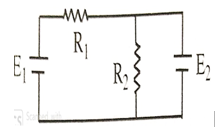Questions

# Two cells of emf E1  and E2 are connected to two resistors  R1 and R2  as shown. If E2   is short circuited then currents through   R1 and R2  areEasy
A

$\frac{{\mathrm{E}}_{1}}{{\mathrm{R}}_{2}},0$

B

$0,\frac{{\mathrm{E}}_{1}}{{\mathrm{R}}_{2}}$

C

$\frac{{E}_{1}}{{R}_{1}},0$

D

$0,\frac{{\mathrm{E}}_{1}}{{\mathrm{R}}_{1}}$